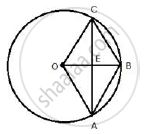Share

# Oabc is a Rhombus Whose Three Vertices A, B and C Lie on a Circle with Centre O. (I) If the Radius of the Circle is 10 Cm, Find the Area of the Rhombus - Mathematics

Course

#### Question

OABC is a rhombus whose three vertices A, B and C lie on a circle with centre O.
(i) If the radius of the circle is 10 cm, find the area of the rhombus

#### SolutionIn rhombus OABC,
OC = 10 cm
∴ OE= 1/2 ×  OB =1/2 × 10 = 5 cm
In Rt. ΔOCE,
OC^2  =  OE^2  + EC^2
⇒ 10^2 =  5^2 +  EC^2
⇒ EC^2 = 100 - 25 =75
⇒ EC =  5sqrt( 3)

∴ AC = 2 xx EC = 2 xx 5sqrt( 3) = 10 sqrt(3)
Area of rhombus = 1/2 xx OB xx AC
= 1/2 xx 10  xx 10sqrt( 3)
=  50 sqrt(3)   cm^2 ≈ 86.6 cm^2 ( sqrt(3) = 1.73)

Is there an error in this question or solution?